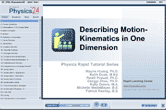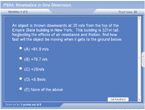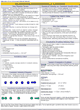How to Learn in 24 Hours?The Rapid Learning Movie

 Need Help? M-F: 9am-5pm(PST): Toll-Free: (877) RAPID-10 US Direct: (714) 692-2900 Int'l: 001-714-692-2900 24/7 Online Technical Support: The Rapid Support Center Secure Online Order:Got Questions? Frequently Asked Questions
 Need Proof? Testimonials by Our Users
 Trustlink is a Better Business Bureau Program. Rapid Learning Center is a fivr-star business. External TrustLink Reviews

 Rapid Learning Courses: MCAT in 24 Hours (2015-16) USMLE in 24 Hours (Boards) Chemistry in 24 Hours Biology in 24 Hours Physics in 24 Hours Mathematics in 24 Hours Psychology in 24 Hours SAT in 24 Hours ACT in 24 Hours AP in 24 Hours CLEP in 24 Hours DAT in 24 Hours (Dental) OAT in 24 Hours (Optometry) PCAT in 24 Hours (Pharmacy) Nursing Entrance Exams Certification in 24 Hours eBook - Survival Kits Audiobooks (MP3)

 Tell-A-Friend: Have friends taking science and math courses too? Tell them about our rapid learning system.Home »  Physics »  College Physics

Describing Motion – Kinematics in One Dimension

 Topic Review on "Title": Kinematics:  The mathematical description of motion without any reference to the cause.  The cause of this motion will be described in a later tutorial. Vector:  A quantity that has magnitude, size, and direction. Velocity and displacement are examples. Scalar:  A quantity that has only magnitude, or size.  Speed, distance, and time are examples. Instantaneous velocity:  The speed of an object at any particular instant. Average velocity: The total distance traveled divided by the total time of travel. Acceleration: The rate of change of velocity.  Acceleration describes how fast an objects speed is changing per amount of time. Kinematic Equations: Formulas that relate the variables of motion. v=d/t a = Δv/Δt=(vf-vi)/t d=vit+at2/2 vf2=vi2+2ad acceleration due to gravity = -9.8 m/s2 Free Fall:  Motion where gravity is the only force acting on an object.  Gravity will accelerate an object at -9.8 meters per second per second.

Rapid Study Kit for "Title":
 Flash Movie Flash Game Flash Card Core Concept Tutorial Problem Solving Drill Review Cheat Sheet"Title" Tutorial Summary : This tutorial describes motion in one direction.  Motion at a constant rate will be discussed.  In this type of motion the velocity remains the same.  Accelerated or changing motion will also be analyzed.  An example of this is a falling object that continually picks up speed as it descends.  The concept of vectors and scalars will be applied.  Various kinematic equations will be used to calculate various quantities in physics problems.  General problem solving hints will be applied to these formulas.

 Tutorial Features: Specific Tutorial Features: • Problem-solving techniques are used to work out the example problems. • Easy explanation for sometimes confusing physics formulas. Series Features: • Concept map showing inter-connections of new concepts in this tutorial and those previously introduced. • Definition slides introduce terms as they are needed. • Visual representation of concepts • Animated examples—worked out step by step • A concise summary is given at the conclusion of the tutorial.

 "Title" Topic List: Constant Velocity Distance Displacement Speed Velocity Average Velocity Constant Velocity Constant Acceleration          Definition          Formula          Units          Free Fall

See all 24 lessons in College Physics, including concept tutorials, problem drills and cheat sheets:
Teach Yourself College Physics Visually in 24 Hours